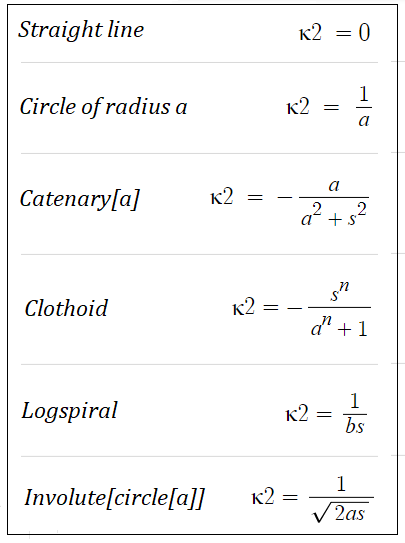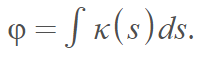# Natural Equation

A natural equation of a curve specifies a curve independent of a choice of coordinate system or parameterization. It relates two properties of the curve: curvature and arc length.For every curve there is a dependence F(s, k) = 0 where s is the arc length and k is the curvature.

The curve is described implicitly, which might not make for easy calculations. However, the natural equation does show how curvature changes with arc length. In addition, the equation is invariant under rotations and translations .

The natural equation arose in the solution to the following space curve problem: Given two one-parameter functions, we want to find a space curve for which the functions are the curvature and torsion (i.e. how the curve twists). Two curves that have the same natural equation also have the same curvature and torsion; they are congruent, only differing by location in the plane .

## Natural Equations for Curves

The natural equation can also be expressed as F(κ2[α], s) = 0, where s is the arc length function of α. This table shows a few natural equations for curvature (κ2) using this notation :The natural equation will often be defined in terms of integrals; Euler was the first to represent the curve in this way to represent special curves — although he didn’t use the term “natural” to describe the equation .Where:

• φ is the angle between the x-axis and the tangent line to the curve,
• κ is the curvature.

We can use this integral to solve the equations for curvature (κ = &kappa(s)) and torsion (τ = 0) with the parametric equations## Natural Equation: Alternate Definitions

In quantum physics, the natural equation is “the pair of Hamilton canonical equations of the total energy function eT” , which can be written asSometimes the term “natural equation” is used colloquially to mean “the most appropriate equation”. For example

“…we extended this result to a variable coefficient case and also to other situations, for example when we have lower order terms in the equation. The most natural equation from stochastic control is the Hamilton-Jacobi-Bellman equation.”

An article on Princeton University’s website  references a natural equation in an article about “beautiful mathematics equations” including Bayes’ theorem and the Dirac equation. However, it seems that this entry, taken in context, may have nothing to do with the equations themselves but may be a synonym for a natural question.## References

 Abbena, E. et al. (2017). Modern Differential Geometry of Curves and Surfaces with Mathematica. CRC Press.
 Knill, O. & Chi, A. (2003). Entry Curves.
 Svetlana, N. et al. (2018). Biometric Inverse Problems. Taylor & Francis.
 Struik, D. (1961). Lectures on Classical Differential Geometry. Addison-Wesley.
 Weisstein, Eric W. “Natural Equation.” From MathWorld–A Wolfram Web Resource. https://mathworld.wolfram.com/NaturalEquation.html
 Crespin, D. The Quantum Captivity of Physics.
 Silvestre, L. (2010). Description of my current research.
 Higham, N. (2016). The Most Beautiful Equations in Applied Mathematics.

CITE THIS AS:
Stephanie Glen. "Natural Equation" From StatisticsHowTo.com: Elementary Statistics for the rest of us! https://www.statisticshowto.com/natural-equation/